# CBSE Sample Papers for Class 12 Computer Science Paper 5

CBSE Sample Papers for Class 12 Computer Science Paper 5 are part of CBSE Sample Papers for Class 12 Computer Science. Here we have given CBSE Sample Papers for Class 12 Computer Science Paper 5.

## CBSE Sample Papers for Class 12 Computer Science Paper 5

 Board CBSE Class XII Subject Computer Science Sample Paper Set Paper 5 Category CBSE Sample Papers

Students who are going to appear for CBSE Class 12 Examinations are advised to practice the CBSE sample papers given here which is designed as per the latest Syllabus and marking scheme, as prescribed by the CBSE, is given here. Paper 5 of Solved CBSE Sample Paper for Class 12 Computer Science is given below with free PDF download Answers.

Time: 3 Hours
Maximum Marks: 70

General Instructions:

• All questions are compulsory within each Section.
• Programming Language in SECTION A : C++.

SECTION A

Question 1.
(a) Find out the reserved keywords which are commonly used in C++ out of the following:
char, Void, virtual, NEW, struct, Throw, auto, iF
(b) Which C++ header file(s) are essentially required to be included to run/execute the following C++ source code?

void main()
{
char Txt;
strcpy(Txt, "COMPUTER");
cout<<Text;
}

(c) Rewrite the following program after removing the syntax error(s), if any. Underline each correction.
Note: Assume all required header files are already being included in the program.

void main()
{
One = 10, Two = 20;
func(0ne;Two);
func(Two);
}
void func(int x, int y = 20)
x = x+y;
cout<>y;
}

(d) Find and write the output of the following C++ program code:
Note: Assume all required header files are already being included in the program.

#include<iostream.h>
struct Company
{
int Salary,Bonus;
};
void Work(Company &C, int N=10)
{
C.Salary++;
C. Bonus += N;
}
void main()
{
Company C = {100, 25};
Work(C, 15);
cout<<C.Salary<<":"<<C.Bonus<<endl;
Work(C);
cout<<C.Salary<<":"<<C.Bonus< Work(C,20);
cout<<C.Salary<<":"<<C.Bonus<<endl:
}

(e) Write the output of the following program:
Note: Assume all required header files are already being included in the program.

class Inc
{
private:
unsigned int count:
public:
Inc()
{
count = 0;
}
void inc_count()
{
count++;
}
int get_count()
{
return count;
}
};
void main()
{
Inc C1, C2;
cout<<"\tC1 = "<<C1.get_count():
cout<<"\tC2 = "<<C2.get_count();
C1.inc_count();
C2.inc_count():
cout<<"\tC1 = "<<C1.get_count():
cout<<"\tC2 = "<<C2.get_count();
}

(f) In the following program, find the correct possible output(s) from the options (i) to (iv) following it. Also, write the maximum and minimum values that can be assigned to variable ‘ToGo’.
Note: Assume all required header files are already being included in the program.
random(n) function generates an integer between 0 to n-1

void main()
{
randomize();
char Name[] = {"Naksh","Saurish","Eashita", "Varun"};
int ToGo;
for(int I=0; I<3; I++)
ToGo = random(2)+1;
cout<<Name[ToGo]<<":";
}
}

Outputs
(i) Saurish: Eashita: Saurish:
(ii) Naksh: Saurish: Eashita:
(iii) Saurish: Eashita: Varun:
(iv) Saurish: Eashita: Eashita:

Question 2.
(a) Differentiate between a data type struct and a data type class in C++.
(b) Observe the following C++ code, answer the questions (i) and (ii).
Note: Assume all necessary header files are included.

class TestMeOut
{
public:
~TestMeOut() //Function1
{
cout<<"Leaving the examination hall”<<endl;
}
TestMeOut() //Function2
{
cout<<"Appearing for examination''<
}
void MyWork() //Function3
{
cout<<"Attempting Questions"<<endl;
}
};

(i) In Object Oriented Programming, what is Functiona1 referred to as and when does it get invoked/called?
(ii) In Object Oriented Programming, what is Function2 referred as and when does it get invoked/called?
(c) Define a class Taxi in C++ with the following description:
Private members

• A data member Taxino of type integer
• A data member Taxiname of type string
• A data member Destination of type string
• A data member Distance of type float
• A data member Fuel of type float
• A member function CALC() to calculate the value of fuel as per the following criteria: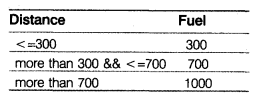Public members

• A function INPUT() to allow the user to enter values for Taxino, Taxiname, Destination, Distance and call function CALC() to calculate the quantity of Fuel.
• A function SHOW() to allow the user to view the content of all the data members.

(d) Answer the questions (i) to (iv) based on the following code:

class Cars
{
char DCode;
protected:
float Price:
void CalcPrice(float);
public:
Cars():
void CInput();
void CShow();
};
class Jeep:public Cars
{
char JName;
float Weight;
public:
Jeep();
void JInput();
void JShow();
};
class ElectronicCars:public Cars
{
char ECName;
char BatteryType;
int Batteries;
public:
ElectronicCars();
void ECInput();
void ECShow();
}

(i) Which type of inheritance out of the following is illustrated in the above example?
I. Single Level Inheritance
II. Multi-Level Inheritance
III. Multiple Inheritance
IV Hierarchical Inheritance
(ii) How many bytes will be required by an object of the class ElectronicCars?
(iii) Write the names of all the data members, which are directly accessible from member functions of the class Jeep.
(iv) Write the names of all member functions, which are directly accessible by an object of the class ElectronicCars.

Question 3.
(a) Write a function void Merge(int A[ ], int B[ ], int C[ ], int n) in C++, which combines the contents of two equi-sized arrays A and B by computing their corresponding elements with the formula 2 * A[i] + 3 * B[i], where value i varies from 0 to n-1 and transfers the resulting content in the third same sized array namely C.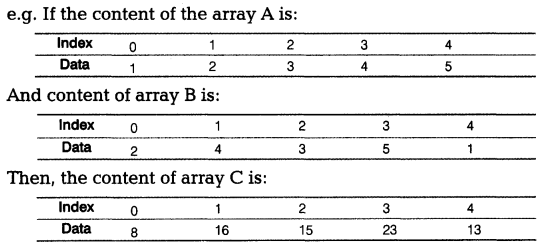(b) An array VAL([1…20] [1…15]) is stored along the row in the memory with each element requiring 4 bytes of storage. If the base address of array VAL is 1500, determine the location of VAL , when the array VAL is stored.
(c) Write a function in C++ to print the sum of all the values, which are either divisible by 4 or are divisible by 5 present in a two dimensional array passed as the argument to the function.
e.g. If the array contains:
5 4 3
6 7 8
10 2 9
Output will be
The sum is: 27
(d) Convert the following infix expression to its equivalent postfix expression showing stack contents for the conversion
(P + Q * (R – S)/T)
(e) Write a function in C++ to perform push operation on a dynamically allocated stack containing real numbers. Consider the following definition
of node in the code:

struct Node
{
float info;
Node * Next;
};
class Stack
{
Node * Top;
public:
stack(); {Top=Null;}
void Push();
void Pop();
~Stack();
};

Question 4.
(a) Find the output of the following C++ code considering that the binary file “s1data.dat” exists on the hard disk with records of 500 members.

class Subject
{
int sid;
char Name;
public:
void Enter();
void Result();
};
void main()
{
fstream f;
f.open("sIdata.dat", ios::binary | ios::in);
Subject S;
int c=0;
while(c<=2)
{
C++;
int POS=f.tellg()/sizeof(S);
cout<<"\nPresent Record:"<<POS<<endl;
f.close();
}

(b) Write a function in C++ to count and display the number of lines that are not starting with alphabets ‘c’ or ‘C’ present in a text file “COM.TXT’. e.g. If the file “COM. TXT” contains the following lines: Computer is must I like computer Students are sitting Come and use computer Characters are not allowed in the password The function should display the output as 2.
(c) Write a function in C++ to add new objects at the bottom of & binary file “COLLEGE.DAT”, assuming the binary file is containing the objects of the following class:

class COLLEGE
{
int Regno:
char Name;
public:
void Input()
{
cin>>Regno;
gets(Name);
}
void Show()
{
cout<<Regno<<Name<
}
};

SECTION B

Question 5.
(a) Observe the following table CLASS and answer the following questions which are asked: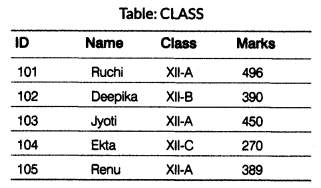(i) Write the most appropriate primary key for the above table and justify your answer.
(ii) What is the degree and the cardinality of the above table?
(b) Consider the following CLUB and COACHES tables. Write SQL queries for (i) to (iv) and find outputs for SQL queries (v) to (viii).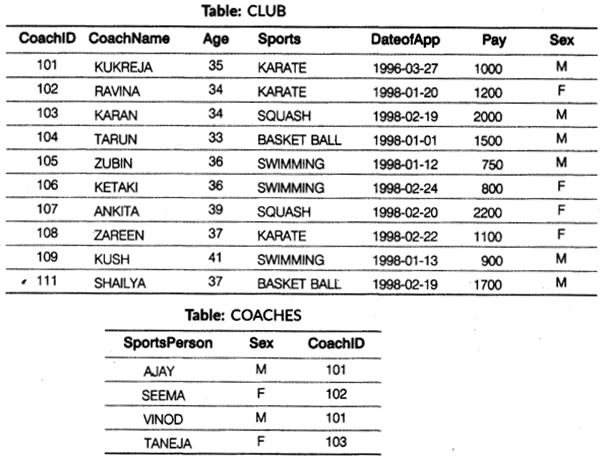To show all information about the swimming coaches in the CLUB.
(ii) To list names of all coaches with their date of appointment (DateofApp) in descending order.
(iii) To display a report showing CoachName, Pay, Age and bonus (15% of pay) for all the coaches.
(iv) To display the CoachName, SportsPerson from table CLUB and COACHES with their nfatching CoachID.
(v) SELECT COUNT(DISTINCT Sports) FROM CLUB;
(vi) SELECT MIN(Age) FROM CLUB WHERE Sex = ‘F’;
(vii) SELECT A.CoachID, A.CoachName, B.SportsPerson FROM CLUB A, COACHES B
WHERE A.CoachID = B.CoachID AND SportsPerson =’VINOD’;
(viii) SELECT CoachName, Age, Sports, SportsPerson, Pay FROM CLUB, COACHES WHERE CLUB.CoachID = COACHES.CoachID AND Pay>1000;

Question 6.
(a) Prove algebraically X.Y + $$\bar { X }$$.Z + Y.Z = X.Y + $$\bar { X }$$.Z
(b) Obtain the Boolean expression for the logic circuit shown below: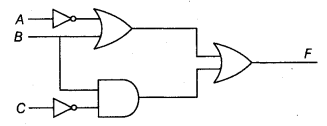(c) Write the POS form of a Boolean function G, which is represented in a truth table as follows: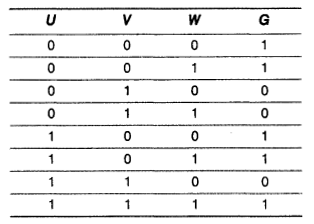(d) Reduce the following Boolean expression using K-map
H(X Y, Z, W) = Σ (0, 1, 2, 3, 5, 7, 8, 9, 10, 14, 15)

Question 7.
(a) What is the important property of circuit switching?
(b) Which protocol provides an error-free connection, which is always faster than the latest conventional modems?
(c) “Mesh topology is excellent for long distance networking”. Justify the statement.
(d) Differentiate between bridge and router.
(e) Give two examples of Web browser and Web server.
(f) Categorize the following under guided media and unguided media:
(i) Bluetooth
(ii) Ethernet
(iii) Infrared
(iv) Fiber
(g) The computer organization has set-up its new branch at Mizoram for its office and Web-based activities. It has 4 wings of building as shown in the diagram.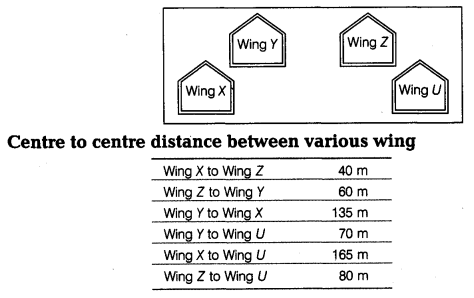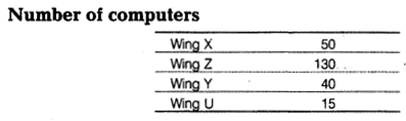(i) Suggest the most suitable cable layout of connections between the wings and topology.
(ii) Suggest the most suitable place (i.e.Wing) to house the server of this organization with a suitable reason with justification.
(iii) Suggest the placement of the following devices with justification:
I. Repeater
II. Hub/Switch
(iv) The organization is planning to link its head office situated in Delhi with the office at Mizoram. Suggest an economical way to connect it, the company is ready to compromise on the speed of connectivity. Justify your answer.

(a) Reserved keywords are char, virtual, struct, auto

(b) (i) → strcpy()
(ii) → cout()

(c) Correct code is:

void func(int x, int y=20);
void main()
{
int One = 10, Two = 20;
func(One, Two);
func(Two);
}
void func(int x, int y)
{
x = x + y;
cout<<x<<y:
}

(d) Output
101 : 40
102 : 50
103 : 70
(e) C1 = 0 C2 = 0 C1 = 1 C2 = 1
(f) The possible outputs are:
(i) Saurish : Eashita : Saurish:
(iv) Saurish: Eashita: Eashita:
The minimum value of ToGo is : 1
The maximum value of ToGo is : 2

(a) Differences between data type struct and data type class are as follows:

 struct data type class data type Data type struct is a logical collection of related dissimilar data items that can be used as a single unit for input/output operation. A class is a collection of not just related data items but also a collection of functions that Operate on those data items. It is composed of only structural constituents. It is composed of structural as well as behavioral constituents. All members of the struct are public by default. All members of the class are private by default. We cannot call a function by using the reference of class. We can call a function by using the reference of class.

(b) (i) The Function1 is referred as destructor and is automatically invoked when the object goes out of scope.
(ii) The Function2 is referred as constructor and is automatically invoked as soon as the object is allocated memory.

(c) class Taxi
{
int Taxino;
char Taxiname;
char Destination;
float Distance,Fuel;
void CALC();
public:
void INPUT():
void SHOW();
};
void Taxi::CALC()
{
if(Distance<=300)
Fuel=300;
else if(Distance<=700)
Fuel=700;
else
fuel=1000;
}
void Taxi::INPUT()
{
cout<<"Enter Taxi number:"; cin>>Taxino;
cout<<"Enter Taxi name:";
gets(Taxiname);
cout<<"Enter Destination:";
gets(Destination);
cout<<"Enter Distance:"; cin>>Distance;
CALC();
}
void Taxi::SHOW()
{
cout<<"Taxi number:"<<Taxino<<endl;
cout<<"Taxi name:"<<Taxiname<<endl;
cout<<"Destination:"<<Destination<<endl;
cout<<"Distance:"<<Distance<<endl;
cout<<"Fuel:"<<Fuel<<endl;
}

(d) (i) IV. Hierarchical Inheritance
(ii) 41 Bytes
(iii) JName, Weight, Price
(iv) ECInput(), ECShow(), Clnput(), CShow()

(a) void Merge(int A[], int B[], int C[], int n)
{
int i;
for(i=0; i<n; i++)
C[i] = 2*A[i]+3*B[i ];
cout<<"Elements of array C are"<<endl;
for(i=0; i<n; i++)
cout<<C[i]<<" ":
}

(b) Base address, B = 1500
Storage, W= 4 bytes
Row Lower Bound, Ir = 1
Row Upper Bound, Ur = 20
Column Lower Bound, Ic = 1
Column Upper Bound, Uc = 15
Number of columns, Nc = 15
The number of rows, NR = 20.
I = 10
J = 9
Using Row Major
VAL [I][J] = B + [(I-Ir) * Nc + (J-Ic)] * W
i.e. VAL  =1500 + [(10-1) * 15 + (9-1)] * 4
= 1500 + (135 + 8) *4
= 1500 + 143 *4
= 1500 + 572
= 2072

(c) void SumArr(int A[]C30], int R, int C)
{
int Sum = 0;
for(int i=0; i<R; i++)
for(int j=0; j<C; j++)
{
if(A[i][j]%4==0 || A[i][j]%5==0)
Sum+=A[i][j];
}
cout<<"The sum is: "<<Sum<<endl;
}

(d) Given expression as (P + Q * {R – S)/T)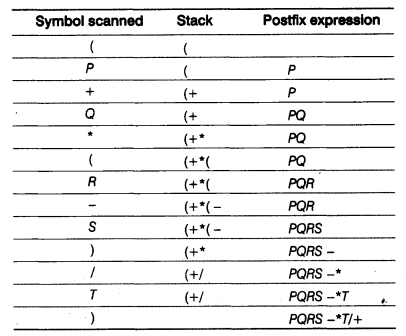So, postfix expression PQRS-*T/+

(e) void Push()
{
Node *Temp;
Temp=new Node;
cout<<"Enter information for new node"; cin>>Temp->info:
if(Top==NULL)
Top=Temp:
else
{
Temp->Next=Top;
Top=Temp:
}
}

(a) Output
Present Record : 3

(b) void CountNonC()
{
ifstream Fin("COM.TXT", ios::in);
char line;
int count=0;
while(!Fin.eof())
{
Fin.getline(line, 255);
if(line!='c' && line!='C')
count++;
}
Fin.close():
cout<<"Total lines not starting with c/C are";
cout<<count<<endl;
}
{
fstream FILE;
FILE.open("COLLEGE.DAT", ios::app | ios::binary);
COLLEGE C;
char ans;
do
{
C.Input();
FILE.write!(char *)&C, sizeof(C));
cout<<"Want to enter more records(Y/N)?"; cin>>ans;
while(ans!='N' && ans!='n');
FILE.close();
}

(a) (i) Primary key is ID because it ensures that a column have an unique identity.
(ii) Degree 4 and cardinality 5

(b) (i) SELECT * FROM CLUB WHERE Sports = "SWIMMING";
(ii) SELECT CoachName FROM CLUB ORDER BY DateofApp DESC;
(iii) SELECT CoachName, Pay, Age, 0.15* Pay AS Bonus FROM CLUB;
(iv) SELECT CoachName, SportsPerson FROM CLUB, COACHES
WHERE CLUB.CoachID=COACHES.CoachID;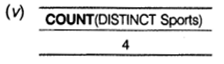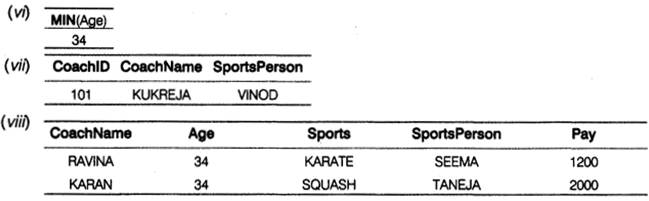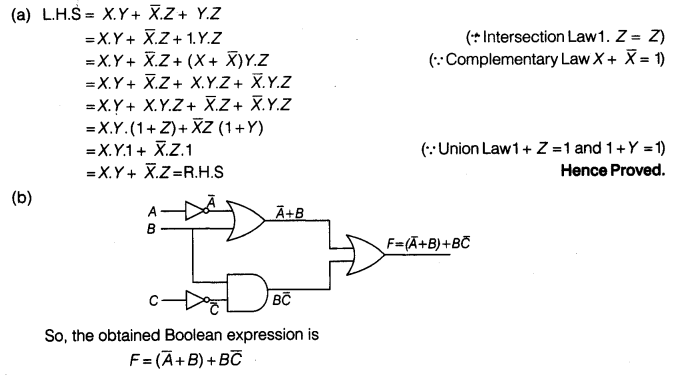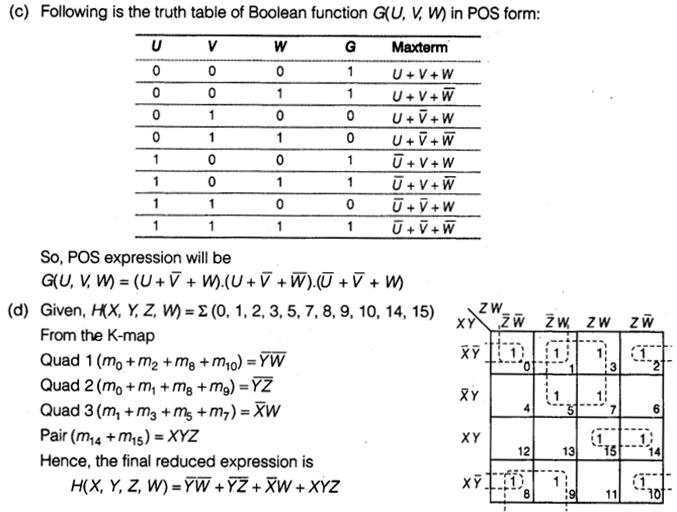(a) The important property of the circuit switching technique is to set-up an end-to-end path (connection) between computers before any data is transmitted.
(b) Telnet
(c) The mesh topology is excellent for long distance networking because it provides extensive back-up, rerouting and pass-through capabilities.
(d) Differences between bridge and router are as follows:

 Bridge Router A bridge connects networks with same standards. A router works like a bridge but can handle different protocols. Bridge uses physical addresses. Router uses logical addresses.

(e) Web browser → Google Chrome, Mozilla Firefox
Web server → Apache HTTP server, Internet Information Services
(f) Guided media
(ii) Ethernet
(iv) Fiber
Unguided media
(i) Bluetooth
(ii) Infrared
(g) (i) Cable Layout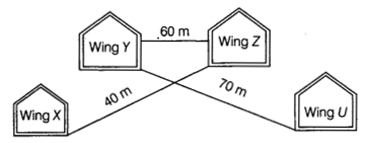(ii) According to the situation, the server can be housed in Wing Zas it has the maximum number of computers, i.e. 130 and if any other wing will house the server the network traffic will be more.
(iii) I. The repeater as per one layout (shown in (i)), the repeater can be avoided as all distance between the wings are <= 100m.
II. HUB/Switch will be required for connecting computers inside each wing since each wing have many computers.
(iv) To connect the head office in Delhi to the office at Mizoram, Wide Area Network (WAN) will be used. Since it has no physical medium but the air channel, no lengthy and expensive cabling system will be required. The connectivity can be done by using satellite transmission since its area coverage is quite large.

We hope the CBSE Sample Papers for Class 12 Computer Science Paper 5 help you. If you have any query regarding CBSE Sample Papers for Class 12 Computer Science Paper 5, drop a comment below and we will get back to you at the earliest.